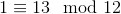# Modular Arithmetic & Congruence Classes

Instructor: Michael Gundlach
Modular arithmetic revolves around remainders; we'll explore what this means in greater detail and learn how to recognize congruence classes. We'll also learn how to add, subtract, and multiply in modular arithmetic.

## Modular (''Clock'') Arithmetic

Have you ever struggled to convert between 12 hour and 24 hour time? Then you're struggling with modular arithmetic. Oftentimes, the trick you'll hear people use to convert from 24 hour time to 12 hour time is to subtract 12 from the time. That is, if the current time is something like 14:23, we subtract 12 from the hour (14) to find that the time is 2:23 pm. We're going to talk about how this works, and how we can both add and multiply numbers using modular arithmetic.

## The Basics

Modular arithmetic is used to measure the distance from an integer to the closest multiple of a particular number, called the modulus. Do you remember the grade school problems where you had to find the remainder after doing a division problem? The remainder is the distance from the dividend (the number being divided) to the divisor (the number we're dividing by). Thus, when doing modular arithmetic, we can add, subtract, and multiply remainders.

To do modular arithmetic, we first need to pick a modulus. For example, we could pick a modulus of 12, which is what we use to tell time. When we divide an integer by this modulus, its remainder is always non-negative and less than the modulus.

For example, when I divide any number by 12, the only possible remainders are the integers 0-11. Because of this, we can represent every integer by its remainder after it's divided by the modulus. This divides the integers into congruence classes, or sets of integers that all have the same remainder when divided by a particular modulus. For example, 1, 13, 25, and 37 all have a remainder of 1 when divided by 12. They are all in the same congruence class when working with a modulus of 12.

When two integers are in the same congruence class for a modulus n, we say they are equivalent modulo n or sometimes just equivalent mod n. For example, 1 is equivalent to 13 modulo 12 since they both have a remainder of 1 when divided by 12. We represent this symbolically by writing the following:We use ≡ rather than = to show that, while the numbers may not be equal on their own, the numbers share an important characteristic; they're equivalent mod n.

## Reducing mod n

Every congruence class has infinitely many members. For example, if it's noon right now, 1 hour from now it'll be 1 o'clock. 13 hours from now, it will also be 1 o'clock. In 25 hours, it will be 1 o'clock again, and so on. Since each congruence class has an infinite number of members, we use the remainder that determines the class to represent the class, just like how all of the times mentioned above were 1 o'clock.

To put it in the language of modular arithmetic, 1, 13, and 25 are all in the same congruence class modulo 12, since they all have a remainder of 1 when divided by 12. We say they are in the congruence class of 1 mod 12. Notice that this means that each congruence class is represented by an integer less than the modulus, since remainders are always less than the modulus.

When given an integer, reducing that integer modulo n means we find the congruence class to which it belongs. For example, if we were to reduce 37 mod 12, we could use long division to determine that the remainder when dividing by 12 is 1.

To unlock this lesson you must be a Study.com Member.

### Register to view this lesson

Are you a student or a teacher?

### Unlock Your Education

#### See for yourself why 30 million people use Study.com

##### Become a Study.com member and start learning now.
Back
What teachers are saying about Study.com

### Earning College Credit

Did you know… We have over 160 college courses that prepare you to earn credit by exam that is accepted by over 1,500 colleges and universities. You can test out of the first two years of college and save thousands off your degree. Anyone can earn credit-by-exam regardless of age or education level.